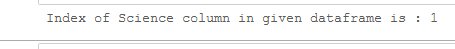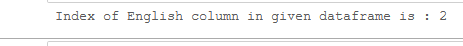# Get column index from column name of a given Pandas DataFrame

In this article we will see how to get column index from column name of a Dataframe. We will use Dataframe.columns attribute and Index.get_loc method of pandas module together.

Syntax: DataFrame.columns

Return: column names index

Syntax: Index.get_loc(key, method=None, tolerance=None)

Return: loc : int if unique index, slice if monotonic index, else mask

Code: Let’s create a Dataframe:

## Python3

 `# import pandas library ` `import` `pandas as pd ` ` `  `# dictionary ` `record ``=` `{``'Math'``: [``10``, ``20``, ``30``,  ` `                   ``40``, ``70``], ` `          ``'Science'``: [``40``, ``50``, ``60``, ` `                      ``90``, ``50``],  ` `          ``'English'``: [``70``, ``80``, ``66``,  ` `                      ``75``, ``88``]} ` ` `  `# create a dataframe ` `df ``=` `pd.DataFrame(record) ` ` `  `# show the dataframe ` `print``(df)`

Output:Dataframe

Example 1: Get a index number of “Science” column.

## Python3

 `# import pandas library ` `import` `pandas as pd ` ` `  `# dictionary ` `record ``=` `{``'Math'``: [``10``, ``20``, ``30``, ``40``, ``70``], ` `          ``'Science'``: [``40``, ``50``, ``60``, ``90``, ``50``],  ` `          ``'English'``: [``70``, ``80``, ``66``, ``75``, ``88``]} ` ` `  `# give column name ` `col_name ``=` `"Science"` ` `  `# find the index no ` `index_no ``=` `df.columns.get_loc(col_name) ` ` `  `print``(``"Index of {} column in given dataframe is : {}"``.``format``(col_name, index_no))`

Output :index number of Science column

Example 2: Get a index number of “English” column.

## Python3

 `# import pandas library ` `import` `pandas as pd ` ` `  `# dictionary ` `record ``=` `{``'Math'``: [``10``, ``20``, ``30``, ` `                   ``40``, ``70``], ` `          ``'Science'``: [``40``, ``50``, ``60``, ` `                      ``90``, ``50``],  ` `          ``'English'``: [``70``, ``80``, ``66``, ` `                      ``75``, ``88``]} ` ` `  `# create a dataframe ` `df ``=` `pd.DataFrame(record) ` ` `  `# give column name ` `col_name ``=` `"English"` ` `  `# find the index no ` `index_no ``=` `df.columns.get_loc(col_name) ` ` `  `print``(``"Index of {} column in given dataframe is : {}"``.``format``(col_name, index_no))`

Output :index number of English column

Attention geek! Strengthen your foundations with the Python Programming Foundation Course and learn the basics.

To begin with, your interview preparations Enhance your Data Structures concepts with the Python DS Course.

My Personal Notes arrow_drop_upCheck out this Author's contributed articles.

If you like GeeksforGeeks and would like to contribute, you can also write an article using contribute.geeksforgeeks.org or mail your article to contribute@geeksforgeeks.org. See your article appearing on the GeeksforGeeks main page and help other Geeks.

Please Improve this article if you find anything incorrect by clicking on the "Improve Article" button below.

Article Tags :

Be the First to upvote.

Please write to us at contribute@geeksforgeeks.org to report any issue with the above content.